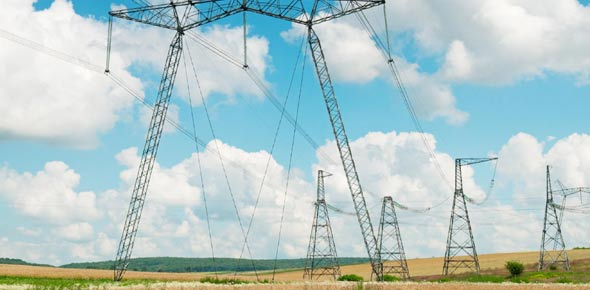# Current Electricity Basic Concept

15 Questions | Total Attempts: 76SettingsComplusory Reflection Quiz. Only attempt when you are ready and confident.

• 1.
Which of the following represent a light bulb?
• A.

I

• B.

Ii

• C.

Iii

• D.

Iv

• 2.
______ is defined as the energy converted from electrical to other forms when one coulomb of electrical charge passes between the two points.
• A.

Current

• B.

Potential difference between any 2 points

• C.

Electromotive force

• D.

Resistance

• 3.
For a solid conductor, what is the name of the particle that carries a chargeand hence creates current call?
• A.

Electron

• B.

Proton

• C.

Neutron

• D.

None of the above

• 4.
This simplified diagram represents a cyclic movement of water where there is a pump, P, to pump water up to a higher level reservoir. The water flows down to the bottom reservoir, which is then again pumped up. Now imagine that this can represent an electric circuit. Which part of the diagram will represent the battery?
• A.

The top reservoir

• B.

The pump

• C.

The piping

• D.

The bottom reservoir

• 5.
This simplified diagram represents a cyclic movement of water where there is a pump, P, to pump water up to a higher level reservoir. The water flows down to the bottom reservoir, which is then again pumped up. Now imagine that this can represent an electric circuit. Identify the positive pole of the “battery” in this diagram
• A.

The left hand side of the top reservoir

• B.

The top part of the pump

• C.

The bottom part of the piping where the water flow from the top reservoir to the bottom

• D.

The right hand side of the bottom reservoir

• 6.
This simplified diagram represents a cyclic movement of water where there is a pump, P, to pump water up to a higher level reservoir. The water flows down to the bottom reservoir, which is then again pumped up. Now imagine that this can represent an electric circuit. What is analogous to the water in an electric circuit?
• A.

Conventional Current

• B.

Electron

• C.

Electromotive force

• D.

Potential difference

• 7.
This simplified diagram represents a cyclic movement of water where there is a pump, P, to pump water up to a higher level reservoir. The water flows down to the bottom reservoir, which is then again pumped up. Now imagine that this can represent an electric circuit. How would you measure the “current” of this “circuit”?
• A.

Measure the rate of water flow

• B.

Measure the weight of the water

• C.

Measure the density of the liquid used

• D.

Measure the amount of energy required by the pump to pump the water up

• 8.
This simplified diagram represents a cyclic movement of water where there is a pump, P, to pump water up to a higher level reservoir. The water flows down to the bottom reservoir, which is then again pumped up. Now imagine that this can represent an electric circuit. Where is the “resistor”?
• A.

The pump

• B.

The bottom reservoir

• C.

The part where the water fall through the pipe

• D.

The end of the pipe where the water gushes out into the bottom reservoir

• 9.
4.0 J of electrical energy is converted to thermal energy when 6.0 C ofcharges pass through a resistor. What is the potential difference across the resistor?
• A.

0.67V

• B.

1.5V

• C.

24V

• D.

Cannot be determined due to lack of information

• 10.
One ______is the p.d. across a load if the work done to bring 1 C ofpositive charge across the load is 1 J.
• A.

Ohm

• B.

Ampere

• C.

Volt

• D.

None of the above

• 11.
To transfer 2.0C of charge from point A to B (where its potentialdifference is 4.0 V) in a circuit. How much energy is needed?
• A.

2.0 J

• B.

6.0 J

• C.

0.50 J

• D.

8.0 J

• 12.
In an electric discharge, a total of 150 C of charges flows into the earth in 1.50 minutes. What is the average current?
• A.

1.67 A

• B.

60.0 A

• C.

100 A

• D.

225 A

• 13.
Which of the flowing constitutes an electric current?I           A flow of electronsII         A flow of positive ionsIII        A lightning discharge
• A.

II only correct.

• B.

I and II are correct.

• C.

II and III are correct.

• D.

I, II and III are correct.

• 14.
The carriers of a current in a wire are electrons. Each electron carries 1.6 x 10-19C of charge. The number of electrons per second passing any section of a wire carrying a current of 0.8 A is
• A.

3 x 10^18

• B.

5 x 10^18

• C.

8 x 10^18

• D.

8 x 10^19

• 15.
The difference in potential between a cloud and earth is 1.0 x 109 V. In a lightning discharge from the cloud to earth, a charge of 20 C passes. Calculate the energy involved in this discharge.
• A.

0.50 x 10^ -8

• B.

0.50 x 10^8

• C.

2.0 x 10^ -8

• D.

2.0 x 10^10

Related TopicsBack to top